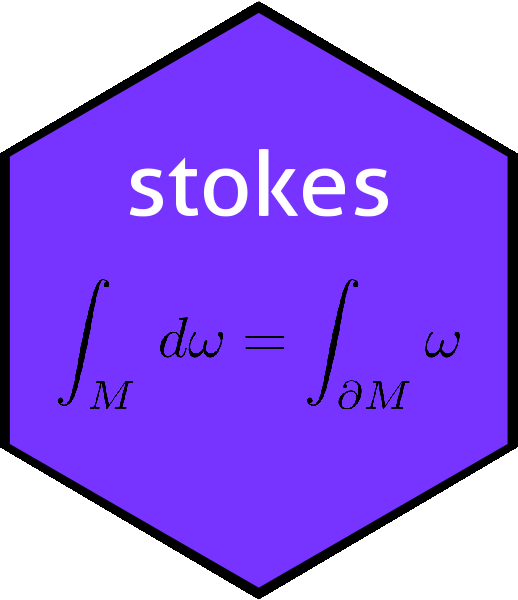# The inner() function in the stokes packageinner
## function (M)
## {
##     ktensor(spray(expand.grid(seq_len(nrow(M)), seq_len(ncol(M))),
##         c(M)))
## }
## <bytecode: 0x560d828dee80>
## <environment: namespace:stokes>

Spivak, in a memorable passage, states:

The reader is already familiar with certain tensors, aside from members of $$V^*$$. The first example is the inner product $$\left\langle{,}\right\rangle\in{\mathcal J}^2(\mathbb{R}^n)$$. On the grounds that any good mathematical commodity is worth generalizing, we define an inner product on $$V$$ to be a 2-tensor $$T$$ such that $$T$$ is symmetric, that is $$T(v,w)=T(w,v)$$ for $$v,w\in V$$ and such that $$T$$ is positive-definite, that is, $$T(v,v) > 0$$ if $$v\neq 0$$. We distinguish $$\left\langle{,}\right\rangle$$ as the usual inner product on $$\mathbb{R}^n$$.

- Michael Spivak, 1969 (Calculus on Manifolds, Perseus books). Page 77

Function inner() returns the inner product corresponding to a matrix $$M$$. Spivak’s definition requires $$M$$ to be positive-definite, but that is not necessary in the package. The inner product of two vectors $$\mathbf{x}$$ and $$\mathbf{y}$$ is usually written $$\left\langle\mathbf{x},\mathbf{y}\right\rangle$$ or $$\mathbf{x}\cdot\mathbf{y}$$, but the most general form would be $$\mathbf{x}^TM\mathbf{y}$$. Noting that inner products are multilinear, that is $$\left\langle\mathbf{x},a\mathbf{y}+b\mathbf{z}\right\rangle=a\left\langle\mathbf{x},\mathbf{y}\right\rangle + b\left\langle\mathbf{x},\mathbf{z}\right\rangle$$ and $$\left\langle a\mathbf{x} + b\mathbf{y},\mathbf{z}\right\rangle=a\left\langle\mathbf{x},\mathbf{z}\right\rangle + b\left\langle\mathbf{y},\mathbf{z}\right\rangle$$ we see that the inner product is indeed a multilinear map, that is, a tensor.

inner(diag(7))
## A linear map from V^2 to R with V=R^7:
##          val
##  6 6  =    1
##  7 7  =    1
##  5 5  =    1
##  3 3  =    1
##  2 2  =    1
##  4 4  =    1
##  1 1  =    1

Note how the rows of the tensor appear in arbitrary order. Verify:

x <- rnorm(7)
y <- rnorm(7)
V <- cbind(x,y)
LHS <- sum(x*y)
RHS <- as.function(inner(diag(7)))(V)
c(LHS=LHS,RHS=RHS,diff=LHS-RHS)
##      LHS      RHS     diff
## 5.503805 5.503805 0.000000

Above, we see agreement between $$\mathbf{x}\cdot\mathbf{y}$$ calculated directly [LHS] and using inner() [RHS]. A more stringent test would be to use a general matrix:

M <- matrix(rnorm(49),7,7)
f <- as.function(inner(M))
RHS <- f(V)
c(LHS=LHS,RHS=RHS,diff=LHS-RHS)
##           LHS           RHS          diff
## -3.410660e+00 -3.410660e+00 -1.332268e-15

(function emulator::quad.3form() evaluates matrix products efficiently; quad.3form(M,x,y) returns $$x^TMy$$). Above we see agreement, to within numerical precision, of the dot product calculated two different ways: LHS uses quad.3form() and RHS uses inner(). Of course, we would expect inner() to be a homomorphism:

M1 <- matrix(rnorm(49),7,7)
M2 <- matrix(rnorm(49),7,7)
g <- as.function(inner(M1+M2))
RHS <- g(V)
c(LHS=LHS,RHS=RHS,diff=LHS-RHS)
##       LHS       RHS      diff
## -5.418253 -5.418253  0.000000

Above we see numerical verification of the fact that $$I(M_1+M_2)=I(M_1)+I(M_2)$$, by evaluation at $$\mathbf{x},\mathbf{y}$$, again with LHS using direct matrix algebra and RHS using inner(). Now, if the matrix is symmetric the corresponding inner product should also be symmetric:

h <- as.function(inner(M1 + t(M1))) # send inner() a symmetric matrix
LHS <- h(V)
RHS <- h(V[,2:1])
c(LHS=LHS,RHS=RHS,diff=LHS-RHS)
##       LHS       RHS      diff
## -22.52436 -22.52436   0.00000

Above we see that $$\mathbf{x}^TM\mathbf{y} = \mathbf{y}^TM\mathbf{x}$$. Further, a positive-definite matrix should return a positive quadratic form:

M3 <- crossprod(matrix(rnorm(56),8,7))  # 7x7 pos-def matrix
as.function(inner(M3))(kronecker(rnorm(7),t(c(1,1))))>0  # should be TRUE
##  TRUE

Above we see the second line evaluating $$\mathbf{x}^TM\mathbf{x}$$ with $$M$$ positive-definite, and correctly returning a non-negative value.

## Alternating forms

The inner product on an antisymmetric matrix should be alternating:

jj <- matrix(rpois(49,lambda=3.2),7,7)
M <- jj-t(jj) # M is antisymmetric
f <- as.function(inner(M))
LHS <- f(V)
RHS <- -f(V[,2:1])   # NB negative as we are checking for an alternating form
c(LHS=LHS,RHS=RHS,diff=LHS-RHS) 
##      LHS      RHS     diff
## 19.50013 19.50013  0.00000

Above we see that $$\mathbf{x}^TM\mathbf{y} = -\mathbf{y}^TM\mathbf{x}$$ where $$M$$ is antisymmetric.